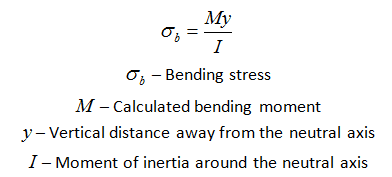# Young's Modulus

• I
Faiq
Suggest why a thin rod can bend more than thick rod without breaking

Flyx
Suggest why a thin rod can bend more than thick rod without breaking

If you look at the equation for stress: F/A, a thicker rod would have more stress for the same force. Thus it would bend less without breaking.

This is just using the equation, I don't know why.

Homework Helper
For the same bending angle, the stress is less in the thinner bar.
The breaking stress is a material characteristic so it is the same for two bars made from the same material.

Faiq
They are asking in terms of extensions (I wrote the same answer and got it wrong)

Homework Helper
Extensions and compressions are also less in a thin rod. They are proportional to the distance from the neutral axis.
But how can be "wrong" to discuss it in terms of stress?

You haven't really explained why the stress is lower in the thinner rod with just that answer. You should really mention that the stress is lower for a given bending angle because the strain is lower, and this is because of the distance from the neutral axis.

•billy_joule
Mentor
The tensile strain at the outside of the bend is equal to the ratio t/R, where t is the distance from the neutral axis and R is the radius of curvature. So, in a thin rod having the same radius of curvature as a thick rod, the distance from the neutral axis at the outside of the bend is less and the tensile strain is less.

DanielSauza
Because the bending stress depends on the distance between it's neutral axis (which is in the center for common shapes in the case of pure bending). So a thicker rod will experience larger maximum bending stress than a slim one when subject to forces of the same magnitude.Mentor
Because the bending stress depends on the distance between it's neutral axis (which is in the center for common shapes in the case of pure bending). So a thicker rod will experience larger maximum bending stress than a slim one when subject to forces of the same magnitude.M/I is the same thing as the elastic modulus E divided by the radius of curvature. So as I said in my post, for the same radius of curvature, with two rods of the same material, the thicker one will have a larger bending stress because it has material elements further from the neutral axis.

hackhard
Suggest why a thin rod can bend more than thick rod without breaking
same reason why a arc of small radius subtends a larger angle at center than a arc of same length and larger radius

Mentor
same reason why a arc of small radius subtends a larger angle at center than a arc of same length and larger radius
Please elaborate on how this answers the OPs question in terms of the stress required to cause the rod to break.